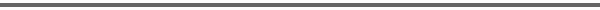Journal Home Page Cumulative Index List of all Volumes Complete Contentsof this Volume Previous Article Next Article Journal of Convex Analysis 10 (2003), No. 1, 275--284 Copyright Heldermann Verlag 2003Automatic Convexity Charles A. Akemann Dept. of Mathematics, University of California, Santa Barbara, CA 93106, U.S.A., akemann@math.ucsb.edu Nik Weaver Dept. of Mathematics, Washington University, St. Louis, MO 63130, U.S.A., nweaver@math.wustl.eduIn many cases the convexity of the image of a linear map with range is Rn is automatic because of the facial structure of the domain of the map. We develop a four step procedure for proving this kind of "automatic convexity". To make this procedure more efficient, we prove two new theorems that identify the facial structure of the intersection of a convex set with a subspace in terms of the facial structure of the original set. Let K be a convex set in a real linear space X and let H be a subspace of X that meets K. In Part I we show that the faces of the intersection of K and H have the form of intersections of F and H for any face F of K. Then we extend our intersection theorem to the case where X is a locally convex linear topological space, K and H are closed, and H has finite codimension in X. In Part II we use our procedure to "explain" the convexity of the numerical range (and some of its generalizations) of a complex matrix. In Part III we use the topological version of our intersection theorem to prove a version of Lyapunov's theorem with finitely many linear constraints. We also extend Samet's continuous lifting theorem to the same constrained siuation. FullText-pdf (294 K)